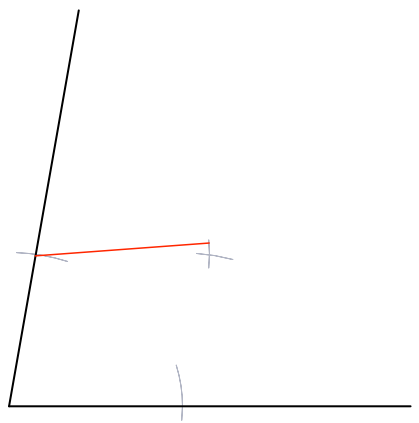Construction - Bisector

## Construction - Bisector

An Angle Bisector splits an angle into two equal parts.

To bisect a given angle, draw an arc, from the point of the angle that intersects both lines.

Keeping the compasses set to the same radius, place the point on each of the intersection points and draw two further arcs, which should cross.

Draw a line from the top of the angle to this point . The angle has now been bisected.

## Example 1

Draw a line at 80º to a base line using a protractor. Bisect the angle wihout using a protractor.

How to do it: draw an angle of 80º using a protractor.

With the point of the compasses on the point of the angle, draw two arcs that will intersect the two lines:From one of the intersection points, create a new arc in the middle of the angle:Repeat from the other intersection point, so that the new arc cuts the arc that has just been drawn:Draw a line from the angle through this new point is the bisector.## Example 2

Using the result of the question, above, draw an angle of 20º.

The previous question bisected the angle of 80º to obtain an angle of 40º.

Repeat the process to bisect one of the 40º angles to obtain an angle of 20º.